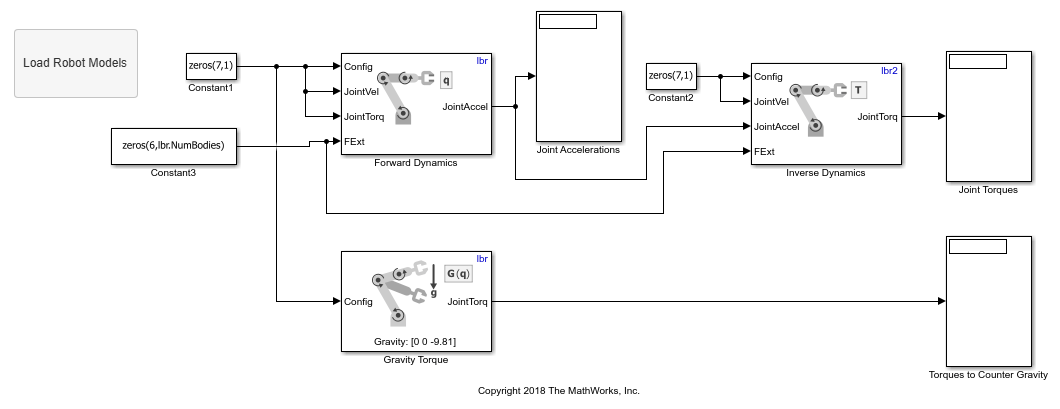# Calculate Manipulator Gravity Dynamics in Simulink

This example shows how to use the manipulator algorithm blocks to compute and compare dynamics due to gravity for a manipulator robot.

Specify two similar robot models with different gravity accelerations. Load the KUKA LBR robot model into the MATLAB® workspace and create a copy of it. For the first robot model, `lbr`, specify a normal gravity vector, `[0 0 -9.81]`. For the copy, lbr2, use the default gravity vector, `[0 0 0]`. These robot models are also specified in the Rigid body tree parameters of the blocks in the model.

```load('exampleLBR.mat','lbr') lbr.DataFormat = 'column'; lbr2 = copy(lbr); lbr.Gravity = [0 0 -9.81]; ```

Open the gravity dynamics model. If needed, reload the robot models specified by the MATLAB code using the Load Robot Models callback button.

```open_system('gravity_dynamics_model.slx') ```The Forward Dynamics block calculates the joint accelerations due to gravity for a given `lbr` robot configuration with no initial velocity, torque, or external force. The Inverse Dynamics block then computes the torques needed for the joint to create those same accelerations with no gravity by using the `lbr2` robot. Finally, the Gravity Torque block calculates the torque required to counteract gravity for the `lbr` robot.

Run the model. Besides some small numerical differences, the gravity torque and the torque required for accelerations due to gravity are the same value with opposite directions.